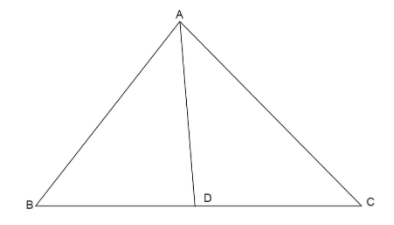QuestionAnswers

# Let $A(2,3,5)$, $B( - 1,3,2)$ and $C(\lambda ,5,\mu )$ be the vertices of a triangle $\vartriangle ABC$. If the median through A is equally inclined to the coordinate axes then:A. $5\lambda - 8\mu = 0$B. $7\lambda - 10\mu = 0$C. $10\lambda - 7\mu = 0$D. $8\lambda - 5\mu = 0$

Hint: Here we use the concept of median, which will give us the midpoint of the base of the triangle where the median meets the base. From point A and the midpoint, we find the direction ratios of the line and since the median is equally inclined to coordinate axes so we take the directional cosines as equal and equating them will give the values of $\lambda ,\mu$.
* Midpoint of a line joining two points $({x_1},{y_1},{z_1}), ({x_2},{y_2},{z_2})$ is given by $\left( {\dfrac{{{x_1} + {x_2}}}{2},\dfrac{{{y_1} + {y_2}}}{2},\dfrac{{{z_1} + {z_2}}}{2}} \right)$
*Directional ratios of a line joining points $P({x_1},{y_1},{z_1}),Q({x_2},{y_2},{z_2})$ is given by
${x_2} - {x_1},{y_2} - {y_1},{z_2} - {z_1}$
* Directional cosines of a line joining points $P({x_1},{y_1},{z_1}), Q({x_2},{y_2},{z_2})$ is given by
$\dfrac{{{x_2} - {x_1}}}{{PQ}},\dfrac{{{y_2} - {y_1}}}{{PQ}},\dfrac{{{z_2} - {z_1}}}{{PQ}}$ where $PQ = \sqrt {{{({x_2} - {x_1})}^2} + {{({y_2} - {y_1})}^2} + {{({z_2} - {z_1})}^2}}$

First we draw a triangle $\vartriangle ABC$, where we draw a median from vertex A to the base BC, the midpoint of BC is D.We find the coordinates of point D using the formula for midpoint of a line.
Midpoint of a line joining two points $({x_1},{y_1},{z_1}), ({x_2},{y_2},{z_2})$ is given by $\left( {\dfrac{{{x_1} + {x_2}}}{2},\dfrac{{{y_1} + {y_2}}}{2},\dfrac{{{z_1} + {z_2}}}{2}} \right)$
Here the points are $B( - 1,3,2), C(\lambda ,5,\mu )$
Mid-point becomes $\left( {\dfrac{{ - 1 + \lambda }}{2},\dfrac{{3 + 5}}{2},\dfrac{{2 + \mu }}{2}} \right)$
So the midpoint of BC is $D\left( {\dfrac{{ - 1 + \lambda }}{2},4,\dfrac{{2 + \mu }}{2}} \right)$.
Now we look at the line joining the points A and D. Since, we have coordinates of the two points, we can write their directional ratios.
Directional ratios of a line joining points $P({x_1},{y_1},{z_1}), Q({x_2},{y_2},{z_2})$ is given by ${x_2} - {x_1},{y_2} - {y_1},{z_2} - {z_1}$
Here points are $A(2,3,5),D(\dfrac{{ - 1 + \lambda }}{2},4,\dfrac{{2 + \mu }}{2})$
So the directional ratios of AD are $(\dfrac{{ - 1 + \lambda }}{2} - 2),(4 - 3),(\dfrac{{2 + \mu }}{2} - 5)$
Taking LCM in the each term
$(\dfrac{{ - 1 + \lambda - 4}}{2}),(1),(\dfrac{{2 + \mu - 10}}{2}) \\ (\dfrac{{\lambda - 5}}{2}),(1),(\dfrac{{\mu - 8}}{2}) \\$
So the directional ratios are $\dfrac{{\lambda - 5}}{2},1,\dfrac{{\mu - 8}}{2}$ … (1)
Since, the median through A is equally inclined to the coordinate axes then it means the directional cosines of the line are equal.
We know directional cosines of a line joining points $P({x_1},{y_1},{z_1}), Q({x_2},{y_2},{z_2})$ is given by
$\dfrac{{{x_2} - {x_1}}}{{PQ}},\dfrac{{{y_2} - {y_1}}}{{PQ}},\dfrac{{{z_2} - {z_1}}}{{PQ}}$ where $PQ = \sqrt {{{({x_2} - {x_1})}^2} + {{({y_2} - {y_1})}^2} + {{({z_2} - {z_1})}^2}}$
Since, the directional cosines are equal we can write
$\dfrac{{{x_2} - {x_1}}}{{PQ}} = \dfrac{{{y_2} - {y_1}}}{{PQ}} = \dfrac{{{z_2} - {z_1}}}{{PQ}}$
Since the length PQ is same in all denominators, we can remove it
${x_2} - {x_1} = {y_2} - {y_1} = {z_2} - {z_1}$ … (2)
From equation (2) we can say the directional ratios of lines are equal, So we equate the directional ratios from equation (1).
$\Rightarrow \dfrac{{\lambda - 5}}{2} = 1 = \dfrac{{\mu - 8}}{2}$
Taking first two terms we get
$\Rightarrow \dfrac{{\lambda - 5}}{2} = 1$
Cross multiplying the values
$\Rightarrow \lambda - 5 = 1 \times 2 \\ \Rightarrow \lambda - 5 = 2 \\$
Shift all constants to one side of the equation
$\Rightarrow \lambda = 2 + 5 \\ \Rightarrow \lambda = 7 \\$
Taking last two terms we get
$\Rightarrow \dfrac{{\mu - 8}}{2} = 1$
Cross multiplying the values
$\Rightarrow \mu - 8 = 1 \times 2 \\ \Rightarrow \mu - 8 = 2 \\$
Shift all constants to one side of the equation
$\Rightarrow \mu = 2 + 8 \\ \Rightarrow \mu = 10 \\$
Now when we take ratio of the values $\lambda ,\mu$
$\Rightarrow \dfrac{\lambda }{\mu } = \dfrac{7}{{10}}$
Cross multiplying the values
$\Rightarrow 10\lambda = 7\mu$
Shift all values to one side of the equation
$\Rightarrow 10\lambda - 7\mu = 0$

So, the correct answer is “Option C”.

Note: Students many times make the mistake of finding the length in the denominator of directional cosines which is not required as the directional cosines are equal. The equal denominators will cancel out when we equate two values. It will make the solution more complex as the length will also be in the form of $\lambda ,\mu$ which are unknown to us.